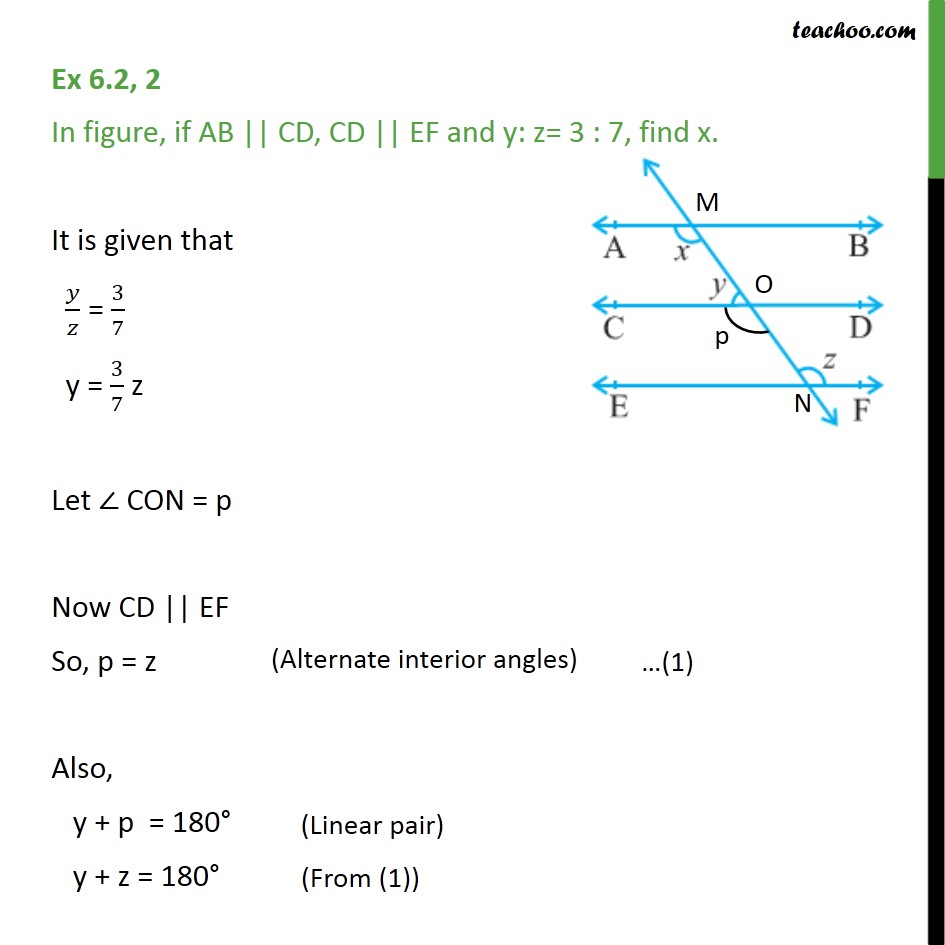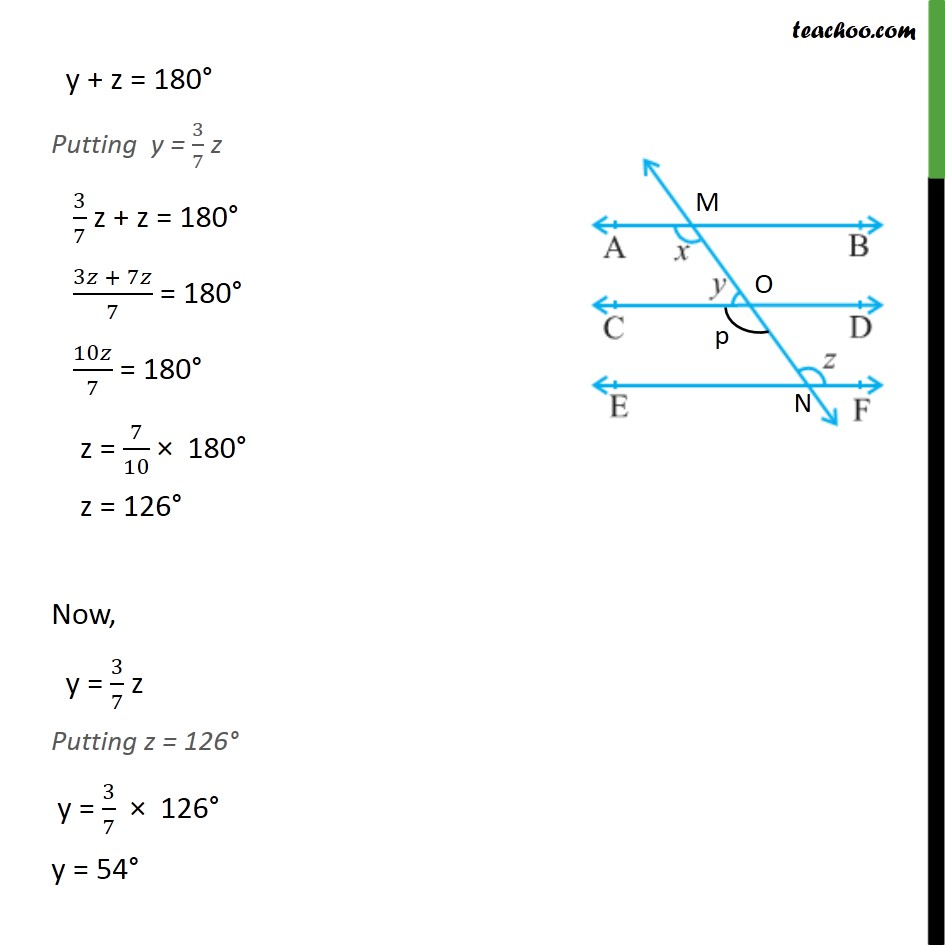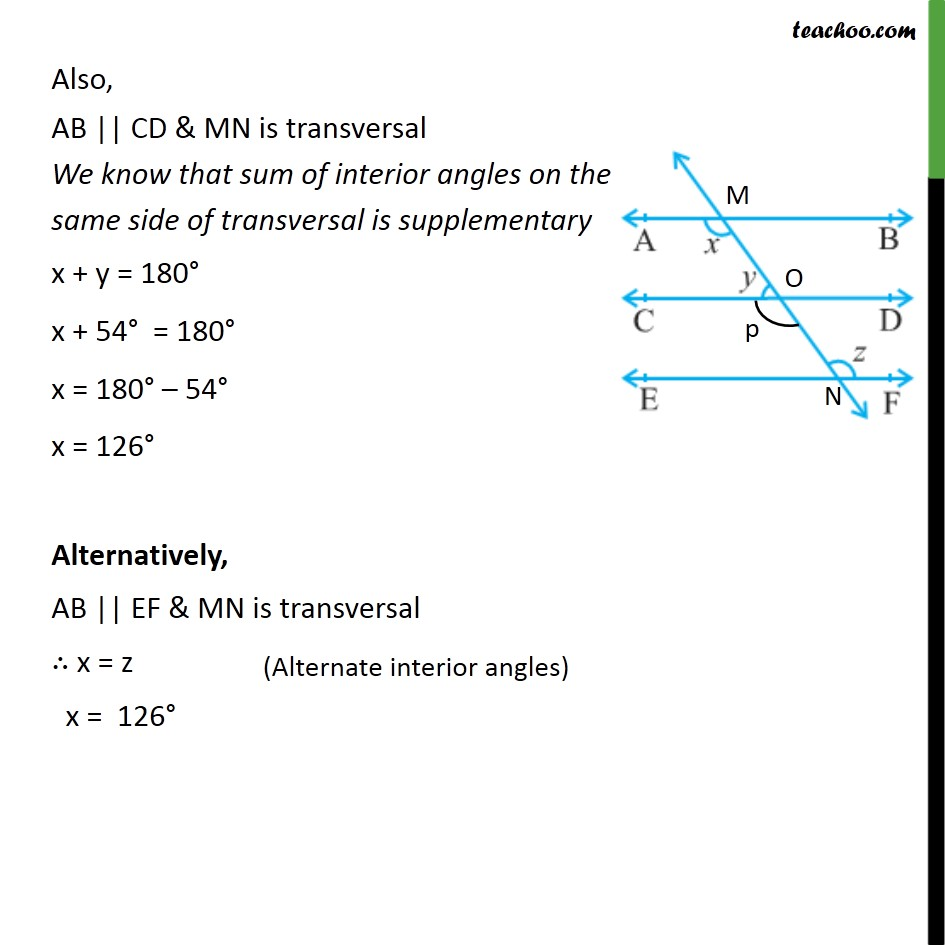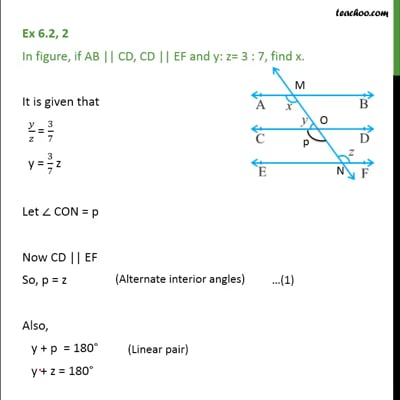Ex 6.2

Chapter 6 Class 9 Lines and Angles
Serial order wiseThis video is only available for Teachoo black users

Solve all your doubts with Teachoo Black (new monthly pack available now!)

### Transcript

Ex 6.2, 2 In figure, if AB || CD, CD || EF and y: z= 3 : 7, find x. It is given that / = 3/7 y = 3/7 z Let CON = p Now CD || EF So, p = z Also, y + p = 180 y + z = 180 y + z = 180 Putting y = 3/7 z 3/7 z + z = 180 (3 + 7 )/7 = 180 10 /7 = 180 z = 7/10 180 z = 126 Now, y = 3/7 z Putting z = 126 y = 3/7 126 y = 54 Also, AB || CD & MN is transversal We know that sum of interior angles on the same side of transversal is supplementary x + y = 180 x + 54 = 180 x = 180 54 x = 126 Alternatively, AB || EF & MN is transversal x = z x = 126